### Introduction to One-Way Analysis of Variance

This is a quick introduction to one-way analysis of variance (ANOVA). The "interludes" scattered along the way can be read after the reader has followed the main line of the argument. Let's begin with a clear statement of what ANOVA is used for:

Given "scores" from samples from several population groups, ANOVA is used to decide whether the differences in the samples' average scores are large enough to conclude that the groups' average scores are unequal. It is a significance test: it begins with the null hypothesis that all the groups have equal averages and that any differences in the sample averages are due to chance in, for example, the way the samples were taken. Then on the basis of this hypothesis, ANOVA computes the value of a variable F; the larger the value of F, the more unlikely it is to have occurred by chance, and hence the more likely that at least one of the populations has an average different from the others. If F is not large enough, the conclusion is that all the populations have equal averages.

Interlude One: The assumptions for ANOVA

To describe what the variable F in ANOVA measures, let's consider two sets of samples from three groups.

Sample data
from
Group
#1:2,2,2,2,3,
3,6,6,6,8
3,3,3,4,4,
4,4,5,5,5
#2:1,1,2,4,5,
5,6,6,7,8,
8,9,9,9,10
4,4,5,5,5,
6,6,6,6,6,
7,7,7,7,9
#3:1,2,2,2,3,
4,5,6,8,8,
9,10,
3,3,4,4,5,
5,5,5,5,6,
7,8

Histograms of samples
from
Group
#1: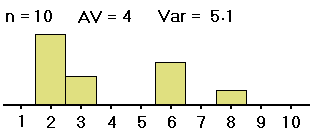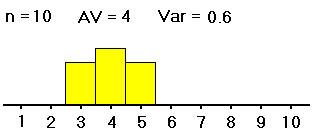#2: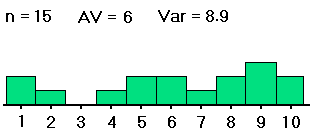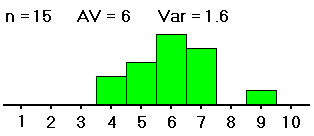#3: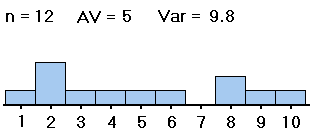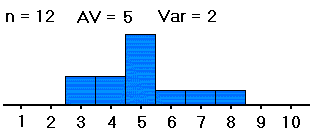(The Var on each histogram is the variance, the square of the SD, so it is a measure of the spread of each sample around its average.)

We want to know whether the averages of groups #1, #2 and #3 -- not the sample averages, which we have computed and are clearly all different, but the averages of the groups as a whole -- are all equal (probably about 5). If the samples were distributed as shown on the left (in both table and histogram), with large spreads about their averages, then small differences between the sample averages would be less noticeable, and less significant, than if the samples were distributed as shown on the right, with the same sample averages but small spreads within the samples. So with data as on the right, we would be more convinced that the group averages are different.

Thus, in order to see whether differences in averages of samples from different groups are significant (i.e., if they indicate that the population groups from which the samples were drawn really have different averages), our computations must include measures of the spreads within the group samples. That is, in deciding whether differences in sample averages are significant, we must also measure variation within the samples, of the data values from their respective sample averages, as well as measuring the spread of the sample averages from the grand average of all the data values. These spreads are related in formulas to yield a measure (the F mentioned above) of the spread of the different sample averages from the grand average versus the spreads within the samples. Here are the formulas:

• Denote the number of groups by I, and use g as the variable running from 1 to I (the number of the group, just used as a label).
• For each group g, denote by ng the number of data values in the sample from that group. And denote by xi,g the i-th data value in the sample from group g (as g runs from 1 to I and i runs from 1 to ng).
• Let N = n1 + n2 + ... + nI, the total number of data values.
• For each group g, denote by AVg the average of the sample from group g, i.e., the sum of the xi,g's (for that fixed g) divided by ng.
• Denote by AV the average of all the data values, i.e. the sum of all the xi,g's divided by N (or, equivalently, the weighted average (n1AV1 + n2AV2 + ... + ngAVg)/N of the sample averages).
• Denote by SSE the sum of the squares (xi,g - AVg)2, as g runs from 1 to I and i runs from 1 to ng. The initials stand for "sum of squared deviations due to error", but it would be better if we understood it as the sum of squared variations of values within the samples from their respective sample averages.
• Denote by SSG the sum of the products ng (AVg - AV)2, which is in essence the sum of the squared differences between the sample averages and the average of all the data points, added once for each data value in the sample. The initials stand for "sum of squared deviations due to groups", i.e., the sum of the squared variations of the sample averages from the overall average (with each squared variation repeated the number of times to represent the size of that sample).
• When we want to decide whether the sample averages from the different groups are significantly different from one another, there are DFE = N-I degrees of freedom in the choice of the individual data values, because we presume that the I sample averages are known; and the "mean squared variation due to error" is the quotient MSE = SSE/DFE. (So the MSE measures the variation within the samples, of the data values from their respective sample averages.)
• And there are DFG=I-1 degrees of freedom in the choice of the sample average from each group, because the overall average is known (as are the sample sizes). The "mean squared variation due to groups" is MSG = SSG/DFG. (So the MSG measures the variation between groups, of the sample averages from the overall average.)
• And finally denote by F the ratio MSG/MSE. Again, the bigger that F is, the more the variation between the group sample averages "dominates" the variation within the samples, and hence the less likely it is that the averages of the (whole) groups are really all equal, and that the differences in the averages of the samples from those groups were just chance.

Interlude Two: Notes on the formulas

Having computed the value of F, we can consult a table for the F-distribution to find the probability (p-value) of getting a value of F at least that large, given the two degrees of freedom (DFE and DFG) determined from the numbers of groups and data values.

Interlude Three: The F-distribution.

The formulas just described are obviously hard to follow, so let us apply them to the two sets of data mentioned earlier:

• In both cases, the number of groups (or of samples) I is 3.
• The three samples on the right contained the same numbers of data values as in the three samples on the left: n1 = 10, n2 = 15 and n3 = 12 on both sides, . . .
• so the sum N of the ng's is the same for both, 10 + 15 + 12 = 37.
Look again at the two sets of histograms:
Histograms of samples
from
Group
#1:#2:#3:• The three sample averages on the left are respectively equal to those on the right: AV1 = 4, AV2 = 6 and AV3 =5 on both left and right.
• Since the sample sizes ng and the sample averages AVg are the same on both sides, so are the overall averages AV (because AV is the average of the AVg's weighted by the ng's): AV = [10(4)+15(6)+12(5)]/37 = 5.1 (approximately).
• Now comes the important part: The SSE is much larger on the left than on the right, because there is more variability within samples, while . . .
• the SSG's are equal, since they depend only on sample averages AVg, overall averages AV and sample sizes ng. (The SSG on both sides is approximately 10(4-5.1)2 + 15(6-5.1)2 + 12(5-5.1)2 = 24.3, while on the left SSE = (1-3)2 + ... [37 terms] = 278 and on the right SSE = (2-3)2 + ... [again, 37 terms] = 54. The SSE's are exact, because the sample averages are whole numbers.)
• The DFE's are equal, N - I = 37 - 3 = 34, and so the MSE is much larger on the left (278/34, or about 8.2, vs. 54/34, or about 1.6, on the right).
• The DFG's are equal, I - 1 = 3 - 1 = 2, and so the MSG's are equal (approximately 24.3/2 = 12.2).
• Thus, because the denominator is smaller on the right, the quotient F = MSG/MSE is much larger on the right (about 12.2/1.6 = 7.7) than on the left (about 12.2/8.2 = 1.5); so the data on the right should have a much smaller, i.e., more significant, p-value. Thus, with the samples on the right, we are more likely to reject the null hypothesis that the groups from which they were taken have equal averages. (In fact, the p-value on the left is not statistically significant, about 24%; while the p-value on the right is highly significant, less than 2/10 of 1%.)

But of course these formulas are evaluated, no longer by hand or even by calculator, but by computer. Indeed, the computer programs not only find the value of F but also find the associated p-value (on the basis of the two values of degrees of freedom), and often give the smallest value of F that would would be needed to yield statistical significance (the "critical F-value". Here are two screen shots from the Excel spreadsheet program, the result of using Excel's built-in ANOVA Single Factor function on the data above. This function is available in the Tools menu under Data Analysis. (If you do not have Data Analysis in your Tools menu, see the first Excel help page linked to our course's index page.) The screens show most of the values mentioned earlier. Also, rather than the "error" vs. "groups" terminology, it uses the clearer "within groups" vs. "between groups".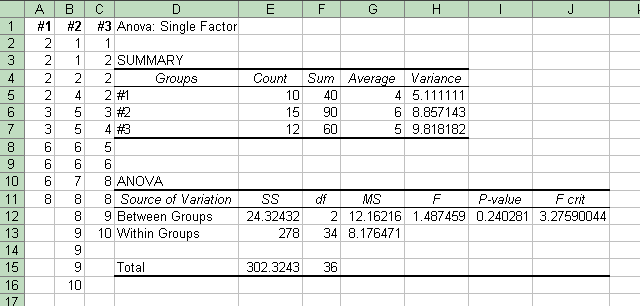This screen shot shows the results of the data shown above on the left side, with large variations within the samples.
 This one shows the results of the data above on the right, with small variations within the samples. Note that many of the values are the same, including "F crit". But the p-value on the right is much smaller; so with the data on the left we do not reject the null hypothesis that the group averages are different; while with the data on the right, we conclude that the averages of groups #1, #2 and #3 are different; the sample averages are not different merely by chance.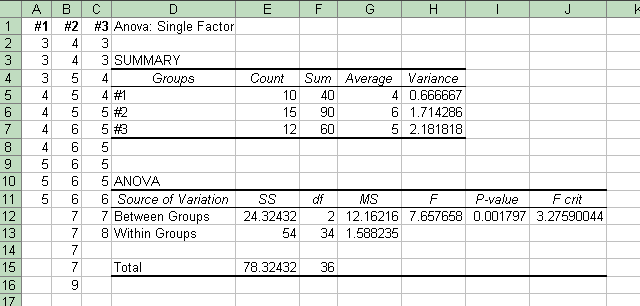Interlude Four: The Source of Variation Total